### Home > CALC > Chapter 1 > Lesson 1.2.2 > Problem1-38

1-38.
1. If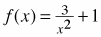, 1-38 HW eTool (Desmos) Homework Help ✎

1. Find the domain and range of f(x).

2. Find expressions for f(−x),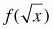, and f(x + h).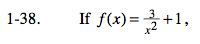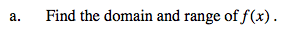Domain: Notice the x in the denominator. What values of x must be excluded?
Range: Notice that x is squared. What does this mean about the outputs of f(x)? Furthermore, what will the +1 do to the range?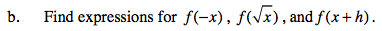$f(\sqrt{x})=\frac{3}{(\sqrt{x})^{2}}+1=\text{\ \ \ \ \ \ \ SIMPLIFY}$

Use the eTool below to view the graphs.
Click the link at right for the full version of the eTool: Calc 1-38 HW eTool